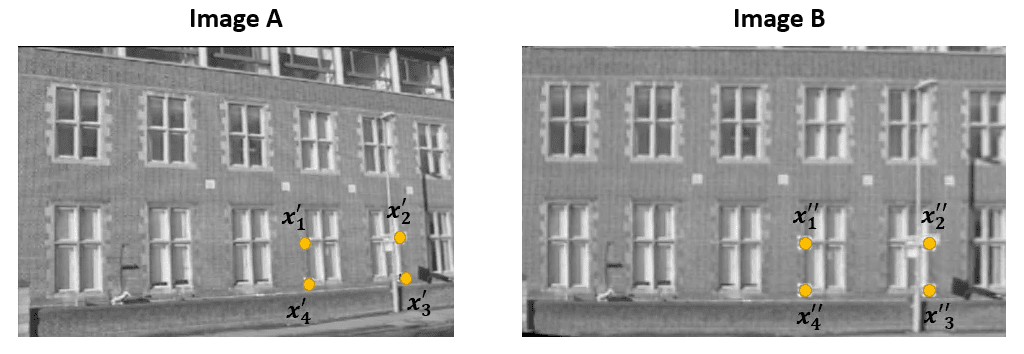## 1. Overview

In this tutorial, we’ll review two important concepts in computer vision, the Fundamental Matrix and the Essential Matrix.

Such matrices play a crucial role in determining the structure and motion of objects in a scene, and their understanding is essential for implementing various computer vision algorithms.

## 2. Mathematics of Fundamental Matrix and Essential Matrix

The fundamental matrix and the essential matrix are two matrices frequently used in stereo geometry whenever one wants to describe geometric relationships between pairs of images either recorded from a stereo camera or a monocular camera moving in the environment.

Suppose we have two images of the same scene and know a set of corresponding points within the two images. For example, we know that pointin image A correspond to the pointsin the image B:The fundamental and essential matrices contain information about the relative orientation of the cameras that can be extracted from corresponding points.

The fundamental and the essential matrices are 3×3 homogeneous matrices with rank 2. Such rank deficiency is used for formulating the so-called coplanarity constraint that can be expressed as:

(1)(2)whereandare the fundamental and the essential matrix,andare the projections of the same point in the two images. The coplanarity constraint equations must hold for all corresponding points.andare generally unknowns, but they can be estimated given a set of corresponding points by solving a system of linear equations. This can be done with the so-called eight-point algorithm, which requires at least 8 corresponding points to estimate the essential or fundamental matrix straightforwardly. The method can work with more than 8 points, and additional points can improve the estimate’s accuracy.

Onceandare known, they can search for correspondences between images.

## 3. Differences

The main difference between the fundamental and the essential matrix is the type of information they encode.

The essential matrixencodes information about the rotation and translation related to the position and orientation of the cameras within the environment.

The fundamental matrixembeds the same information asand contains information about the intrinsic parameters of both cameras. In other words, the matrixis a purely geometric entity that has no connection to imagers properties. It maps the location of a pointin physical coordinates, as seen by the left camera, to the projection of the same point, as seen by the right camera.

On the other hand, the fundamental matrixrelates the location of the point in the two images using image coordinates (pixels). Therefore, the fundamental matrix is used for uncalibrated cameras, while the essential matrix is used for calibrated cameras.

Another difference is the number of degrees of freedom (DoF).has 7 DoF, whilehas 5 DoF since it considers the cameras’ intrinsic parameters.

## 4. Application

Here are some applications of the essential and fundamental matrix.

• Structure from Motion: the process of reconstructing the three-dimensional structure of a scene from multiple 2D images. Given a sequence of images captured by a moving camera, the essential matrix can be used to estimate the relative orientation and position of the cameras. This information can then be used to triangulate the three-dimensional structure of the scene, allowing us to create a 3D reconstruction of the scene
• Camera Calibration: the fundamental matrix can be used to estimate the intrinsic parameters of the cameras, such as the focal length and principal point. Camera calibration allows for correcting distortions due to the camera lens, i.e. radial distortion and tangential distortion
• Image Rectification: the transformation used to project images captured by a stereo camera onto a common image plane. The fundamental matrix can be used to determine the homography that transforms the images into a rectified form. Image rectification simplifies the information extraction of the three-dimensional structure of the scene

## 5. Conclusion

In this article, we reviewed the fundamental matrix and the essential matrix, two important tools in computer vision for determining the relationships between two images of a scene. We discussed their differences and provided their main applications in computer vision.